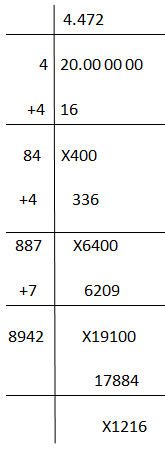# Square Root of 20

The value of the square root of 20 is 4.472135955 and is denoted as √20 in radical form. This value we can easily get from the calculator. But, students should know how to simplify the root of any number without using a calculator. This is possible by using two methods: Prime factorisation and long division method. Both methods are very simple and are easy ways to find the square root.

 Square root of 20, √20 = 4.4721 (in round figures)

The square root of 20 is represented as √20, where √ is the radical sign and 20 is said to be radicand. In the simplest form, the √20 can be written as 2√5. Therefore, here we cannot say that √20 is a rational number since √5 is an irrational number.

Now, let us learn here to find the value of √20.

## Simplification of Square Root of 20

The simple method to find the value of √20 is to factorise the number under the root, i.e. 20.

The prime factorisation of 20 gives:

20 = 2 x 2 x 5 = 22 x 5

Thus, here we can see, 20 is not a perfect square, because we cannot pair digit 5 after factoring 20. Only 22 can be taken out of the root.

Hence, if we take the roots on both sides, we get;

√20 = √(2 x 2 x 5)

Here, we just have only one pair of numbers, which is 2. Therefore, taking it outside the root, we get;

√20 = 2√5

From the square root table, we know the value of √5 = 2.236

Hence,

√20 = 2 x 2.236

√20 = 4.472

### Using Long Division Method

We have learned to calculate the value of the square root of 20 using prime factorisation. But it is not a direct method to find the value because 20 is an imperfect square and we need to remember the √5 value to find the accurate value of √20.

By using long division method, we can use the accurate value of √20. Below is the solved step presented to evaluate √20.## Video Lessons on Square Roots

### Visualising square roots### Finding Square rootsFind more square roots here:

Test your Knowledge on Square Root of 20